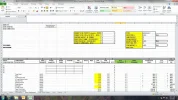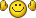# Loop Repeats - PLS HELP

#### Alcaide1

Hi, I have a loop that continues to repeat itself. I am new at this Macro stuff. Problem seems to be where I want to remove lines w/o quantity in the section "Remove all rows that have No Quantity". can anyone point me in the right direction?

Dim RowNumber As Integer
Dim FirstRow As Integer
Dim Financial As Variant

Financial = ActiveWorkbook.Name

' Find out Row Number for Headings
Sheets("Price Worksheet").Select
Range("A17").Select
RowNumber = InputBox("What Number Row are the x's in (gray row) ?", "HEADINGS ROW", 17)
FirstRow = RowNumber + 4
' Unhide the Summary Template & get Some Values
Dim Customer As String
Dim Quotation As String

Sheets("Summary Template").Visible = True
Sheets("Summary Template").Select
Sheets("Summary Template").Copy Before:=Sheets("Summary Template")
Sheets("Summary Template (2)").Select
Sheets("Summary Template (2)").Name = "SUMMARY"
Sheets("Summary Template").Visible = False

Sheets("SUMMARY").Activate

Customer = Range("B1").Value
Quotation = Range("B2").Value

Range("A13").Activate
Sheets("Price Worksheet").Activate

' Find out Which Columns need to be added to Summary
Dim N As Integer
Dim NewRange As Range
Dim Cell As Range
Dim X As Integer

N = 1
X = 0

For Each Cell In Range("MarkBox")

Sheets("Price Worksheet").Activate
If Cell.Value = "X" Or Cell.Value = "x" Then
Range("A" & RowNumber).Activate
ActiveCell.Offset(1, X).Range("A1:A1500").Select
Selection.Copy
'If Cells(RowNumber, N).Value = "x" Then
' Cells(FirstRow, N).Copy

Sheets("SUMMARY").Activate
ActiveCell.PasteSpecial xlPasteValuesAndNumberFormats
ActiveCell.Offset(0, 1).Select

End If

N = N + 1
X = X + 1

Next Cell
' Copy & Save Summary to New book
Sheets("SUMMARY").Select
Sheets(Array("SUMMARY", "Notes")).Copy

SaveFile:
SaveAsFile = Application.GetSaveAsFilename(Customer & " - " & Quotation & " - SUMMARY.xls", "Excel files, *.xls", 1, "Select your folder & Filename")

If SaveAsFile <> "False" Then
ActiveWorkbook.SaveAs SaveAsFile, FileFormat:=xlNormal
End If

If SaveAsFile = "False" Then
MsgBox ("You must save Price Schedule with new file name!!!")
GoTo SaveFile

End If
SummaryBook = ActiveWorkbook.Name

' Value out top section & remove links
Sheets("SUMMARY").Select
Rows("1:11").Select
Selection.Copy
Selection.PasteSpecial xlPasteValues

Range("A1").Select

' Remove all rows that have NO quantity

Dim R As Integer
R = 16
QTYCOL = InputBox("What column is QTY in on new sheet?", "QTY Col", "G")

Do Until Range(QTYCOL & R).Value = "XXX"
If Range(QTYCOL & R).Value < 1 Then
Rows(R).Delete

R = R - 1

End If

R = R + 1

Loop

' Insert rows before & after TOTALS
Cells.Select
Selection.Find(What:="DESCRIPTIONS").Activate

Do Until ActiveCell.Value = "XXX"
ActiveCell.Offset(1, 0).Range("A1").Select
If Left(ActiveCell, 5) = "TOTAL" Then
'Selection.EntireRow.Font.Bold = True
Selection.EntireRow.Insert
ActiveCell.Offset(2, 0).Range("A1").Select
Selection.EntireRow.Insert
End If
Loop

ActiveCell.Offset(1, 0).Range("A1").Select

' Make TOTAL ROWS BOLD & COLOR
Range("A14:AC14").Select
Selection.Find(What:="DESCRIPTIONS").Activate

Do Until ActiveCell.Value = "XXX"
ActiveCell.Offset(1, 0).Range("A1").Select
If Left(ActiveCell, 5) = "TOTAL" Then
Selection.EntireRow.Font.Bold = True
ActiveCell.Interior.Color = vbYellow
End If

If ActiveCell.Value = "GRAND TOTAL" Then
Selection.EntireRow.Font.Bold = True
ActiveCell.Interior.Color = vbGreen
End If

Loop

ActiveCell.Offset(1, 0).Range("A1").Select
' Delete the XXX's
Cells.Find(What:="XXX").Activate
Selection.EntireRow.Delete
' Delete Summary tab from workbook
Workbooks(Financial).Activate
Sheets("Summary").Delete
Workbooks(SummaryBook).Activate

End Sub

#### Attachments

•Excel1.jpg
190.9 KB · Views: 268

#### Sifou

Hi,

I am not sure how VBA interprets your range ((Range(QTYCOL & R).Value) to be honest as I use VBA from time to time to help friends using excel as their main tool.

However as you said you seem to be new in VBA, it might be good for you to know that there is a debugger in the Visual Basic Editor which you can use to stop at a given point in the code and evaluate the value and/or properties of any cell, variable, object referenced in your code.

for your code to stop you have to use a breakpoint, which you can add on a line of your choice by putting the cursor on that line and pressing F9, your code will now stop on the line everytime this line will run, therefore once everytime you run the macro for a regular line or several times if the line is within a loop.

In your situation try to put a breakpoint on the line starting "If Range(QTYCOL &R...".

Then the debugger will allow you to evaluate object at runtime, to see the value or properties of an object or a variable you can add what we call a watch, by selecting the object to evaluate (here i would select Range(QTYCOL & R).Value) and then by right clicking on the selection and choosing Add Watch. at this point run your macro, it should stop on your breakpoint and you can start troubleshooting you issue by debugging your VBA macro. let me know if that helped. Thanks

also i just changed the way you increment the number of rows R so that it s easy to read (i.e. we increment only for row where value is greater than 1):

Do Until Range(QTYCOL & R).Value = "XXX"
If Range(QTYCOL & R).Value < 1 Then
Rows(R).Delete
else
R = R + 1
End If
Loop

#### Alcaide1

Hi Sifou,
I am very new to VBA lol. I tried your fix, but it continues to loop w/o stopping still. Any chance we can work on this offlilne? My personal email address is (e-mail address removed)
Please email me your contact info. and I will send you a file. I appreciate your help.